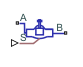# Gate Valve (IL)

Gate valve in an isothermal liquid system

Since R2020a

•Libraries:
Simscape / Fluids / Isothermal Liquid / Valves & Orifices / Flow Control Valves

## Description

The Gate Valve (IL) block represents flow control by a gate valve in an isothermal liquid network. The valve comprises a round, sharp-edged orifice and a round gate with the same diameter. The gate opens or closes according to the displacement signal at port S. A positive signal retracts the gate and opens the valve.

### Opening Area

Gate Valve Opening SchematicThe area open to flow as the gate retracts is:

`${A}_{open}=\frac{\pi {d}_{0}^{2}}{4}-{A}_{shielded},$`

where d0 is the Valve orifice diameter. The area shielded by the gate in a partially open valve is:

`${A}_{shielded}=\frac{{d}_{0}^{2}}{2}{\mathrm{cos}}^{-1}\left(\frac{\Delta l}{{d}_{0}}\right)-\frac{\Delta l}{2}\sqrt{{d}_{0}^{2}-{\left(\Delta l\right)}^{2}},$`

where Δl is the gate displacement, which is the sum of the signal at port S and the Gate position when fully covering orifice.

If displacement exceeds the Orifice diameter, the valve area Aopen is the sum of the maximum orifice area and the Leakage area:

`${A}_{open}=\frac{\pi }{4}{d}_{0}^{2}+{A}_{leak}.$`

For any combination of the signal at port S and the gate offset that is less than 0, the minimum valve area is the Leakage area.

### Numerically Smoothed Displacement

When the valve is in a near-open or near-closed position, you can maintain numerical robustness in your simulation by adjusting the parameter. If the parameter is nonzero, the block smoothly saturates the gate displacement between `0` and the Valve orifice diameter parameter. For more information, see Numerical Smoothing.

### Mass Flow Rate Equation

Mass is conserved through the valve:

`${\stackrel{˙}{m}}_{A}+{\stackrel{˙}{m}}_{B}=0.$`

The mass flow rate through the valve is calculated as:

`$\stackrel{˙}{m}=\frac{{C}_{d}{A}_{valve}\sqrt{2\overline{\rho }}}{\sqrt{P{R}_{loss}\left(1-{\left(\frac{{A}_{valve}}{{A}_{port}}\right)}^{2}\right)}}\frac{\Delta p}{{\left[\Delta {p}^{2}+\Delta {p}_{crit}^{2}\right]}^{1/4}},$`

where:

• Cd is the Discharge coefficient.

• Avalve is the valve open area.

• Aport is the Cross-sectional area at ports A and B.

• $\overline{\rho }$ is the average fluid density.

• Δp is the valve pressure difference pApB.

The critical pressure difference, Δpcrit, is the pressure differential associated with the Critical Reynolds number, Recrit, which is the flow regime transition point between laminar and turbulent flow:

`$\Delta {p}_{crit}=\frac{\pi \overline{\rho }}{8{A}_{valve}}{\left(\frac{\nu {\mathrm{Re}}_{crit}}{{C}_{d}}\right)}^{2}.$`

Pressure loss describes the reduction of pressure in the valve due to a decrease in area. PRloss is calculated as:

`$P{R}_{loss}=\frac{\sqrt{1-{\left(\frac{{A}_{valve}}{{A}_{port}}\right)}^{2}\left(1-{C}_{d}^{2}\right)}-{C}_{d}\frac{{A}_{valve}}{{A}_{port}}}{\sqrt{1-{\left(\frac{{A}_{valve}}{{A}_{port}}\right)}^{2}\left(1-{C}_{d}^{2}\right)}+{C}_{d}\frac{{A}_{valve}}{{A}_{port}}}.$`

Pressure recovery describes the positive pressure change in the valve due to an increase in area. If you do not wish to capture this increase in pressure, clear the Pressure recovery check box. In this case, PRloss is 1.

## Ports

### Input

expand all

Physical signal port associated with the valve gate displacement, in m. A positive signal retracts the gate and opens the valve.

### Conserving

expand all

Isothermal liquid conserving port associated with valve inlet A.

Isothermal liquid conserving port associated with valve inlet B.

## Parameters

expand all

Valve open-area diameter.

Gate offset when the valve is closed. A positive, nonzero value indicates a partially open valve when the signal at port S is 0. A negative, nonzero value indicates an overlapped valve that remains closed for an initial positive displacement set by the physical signal at port .

Sum of all gaps when the valve is in the fully closed position. Any area smaller than this value is saturated to the specified leakage area. This contributes to numerical stability by maintaining continuity in the flow.

Areas at the entry and exit ports A and B, which are used in the pressure-flow rate equation that determines the mass flow rate through the orifice.

Correction factor accounting for discharge losses in theoretical flows.

Upper Reynolds number limit for laminar flow through the valve.

Continuous smoothing factor that introduces a layer of gradual change to the flow response when the valve is in near-open or near-closed positions.

Whether to account for pressure increase when fluid flows from a region of smaller cross-sectional area to a region of larger cross-sectional area.

## Version History

Introduced in R2020a